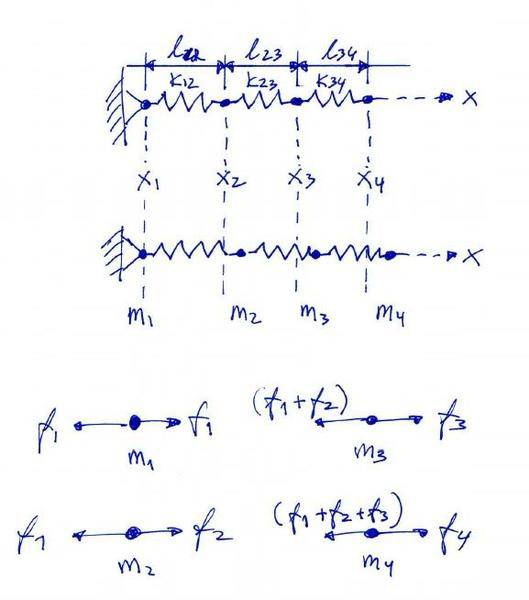# Coupled mass problem

Dafe

## Homework Statement

Suppose masses $$m_{1}, m_{2}, m_{3}, m_{4}$$ are located at positions $$x_{1}, x_{2}, x_{3}, x_{4}$$ in a line and connected by springs with constants $$k_{12}, k_{23}, k_{34}$$ whose natural lengths of extension are $$l_{12}, l_{23}, l_{34}$$.
Let $$f_{1}, f_{2}, f_{3}, f_{4}$$ denote the rightward forces on the masses, e.g.,
$$f_{1} = k_{12}(x_{2} - x_{1} - l_{12})$$

a) Write the 4 X 4 matrix equation relating the column vectors $$f$$ and $$x$$. Let $$K$$ denote the matrix in this equation.

## The Attempt at a Solution

I'm trying to find the rightward force acting on every mass as the springs are stretched.

$$f_{2} = k_{23}(x_{3} - x_{2} - l_{23}) - f_{1}$$
$$f_{3} = k_{34}(x_{4} - x_{3} - l_{34}) - (f_{1} + f_{2})$$
$$f_{4} = f_{1} + f_{2} + f_{3}$$

It seems quite complicated to put this into matrix form, so I'm assuimg that I've done something wrong.
Suggestions?

Dafe
By the way, here is a figure showing how I am visualizing this problem:
I am assuming $$m_{1}$$ to be constrained. Just throwing out ideas :)Last edited:
Dafe
Here's my complete solution. I expand and simplify the equations given in my first post.
Then I put together expressions for the x's and separate the constants.

$$$f_{1} = k_{12}(x_{2}-x_{1}-l_{12}) = k_{12}x_{2} - k_{12}x_{1} - k_{12}l_{12}$ $k_{12}l_{12} = const.$ $f_{2} = k_{23}(x_{3}-x_{2}-l_{23} - k_{12}(x_{2}-x_{1}-l_{12}))$ $\Rightarrow f_{2} = x_{2}(-k_{12}-k_{23}) + x_{1}k_{12} + x_{3}k_{23} - k_{23}k_{23} + k_{12}l_{12}$ $- k_{23}k_{23} + k_{12}l_{12} = const.$ $f_{3} = k_{34}(x_{4}-x_{3}-l_{34}) - k_{23}(x_{3}-x_{2}-l_{23})$ $\Rightarrow f_{3} = k_{23}x_{2} + x_{3}(-k_{23}-k_{34}) + k_{34}x_{4} -k_{34}l_{34} + k_{23}l_{23}$ $-k_{34}l_{34} + k_{23}l_{23} = const.$ $f_{4} = k_{34}(x_{4}-x_{3}-l_{34}) = k_{34}x_{4} - k_{34}x_{3} - k_{34}l_{34}$ $- k_{34}l_{34} = const.$ $\textbf{f} = \textbf{K}\textbf{x} + \textbf{c}$ $\left[\begin{array}[pos]{c} f_{1} \\ f_{2} \\ f_{3} \\ f_{4} \\ \end{array}\right]= \left[\begin{array}[pos]{cccc} -k_{12} & k_{12} & 0 & 0 \\ k_{12} & (-k_{12}-k_{23}) & k_{23} & 0 \\ 0 & k_{23} &(-k_{23}-k_{34}) & k_{34} \\ 0 & 0 &-k_{34} & k_{34}\\ \end{array}\right] \left[\begin{array}[pos]{c} x_{1} \\ x_{2} \\ x_{3} \\ x_{4} \\ \end{array}\right]+ \left[\begin{array}[pos]{c} -k_{12}l_{12} \\ -k_{23}l_{23} + k_{12}l_{12} \\ -k_{34}l_{34} + k_{23}l_{23} \\ -k_{34}l_{34} \\ \end{array}\right]$$$